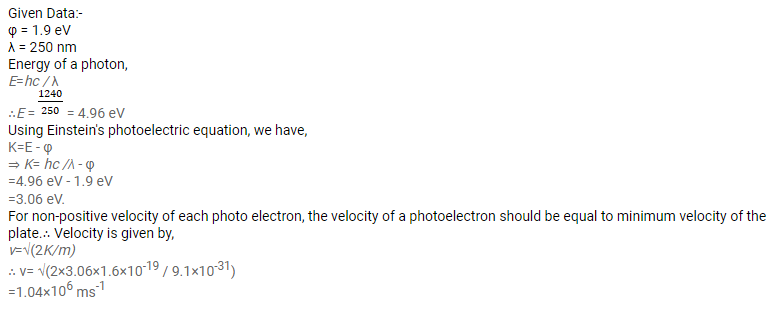# A horizontal cesium plate (φ = 1.9 eV) is moved vertically downward

Question:

A horizontal cesium plate $(\varphi=1.9 \mathrm{eV})$ is moved vertically downward at a constant speed $v$ in a room full of radiation of wavelength $250 \mathrm{~nm}$ and above. What should be the minimum value of $v$ so that the vertically-upward component of velocity is non-positive for each photoelectron?

Solution: